Latest articles
• Symfony
• Others
• Others
• Java
• Java

# How to find nearest locations from a collection of coordinates (latitude and longitude) with PHP, MySQL

One of the most popular features that a lot of applications are offering nowadays, is the possibility to locate the nearest registers from a database given a custom position (your current position maybe, specifying the latitude and longitude). For example, in the real estate business, is a pretty useful implementation, if you could simply place yourself in the center of a city, for example New York and the application will allow you to display in the maps the closest available houses/apartments in a radius of 50/100 KM: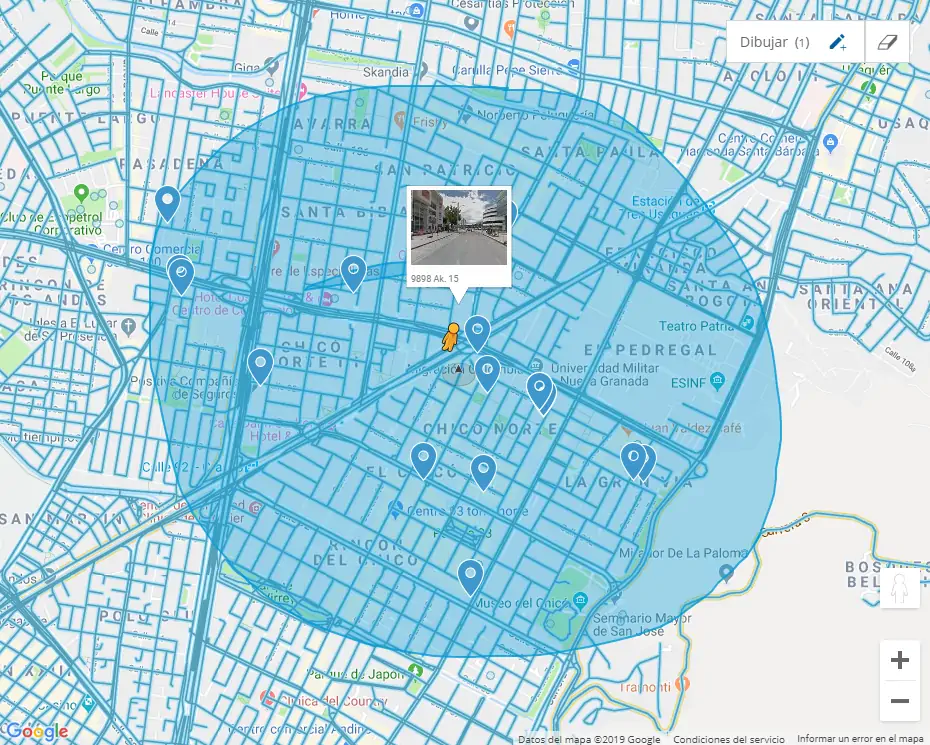The idea by itself is pretty cool, right? Obviously, we are not including places from Google Maps, that means that the markers will be ours obviously and they are stored in our own database. In this article, we will explain you how to know the nearest location from a collection of coordinates in MySQL.

## 1. Understanding our markers database

To get started, you will obviously need locations stored in your database, in our case we will have a very simple database structure so it can be easily understood by everyone. The database can be created with the following query:

``````CREATE TABLE `markers` (
`id` bigint(20) NOT NULL,
`lat` decimal(10,8) NOT NULL,
`lng` decimal(11,8) NOT NULL,
`name` varchar(255) CHARACTER SET utf8 NOT NULL
) ENGINE=InnoDB DEFAULT CHARSET=utf8;``````

It simply stores markers that are identified by a name and contain the coordinates of latitude and longitude. It contains the following data: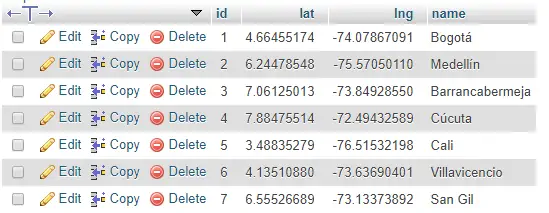If we place the markers of the mentioned locations in Google Maps using JavaScript:

``````var markers = [
{
"id": "1",
"lat": "4.66455174",
"lng": "-74.07867091",
"name": "Bogot\u00e1"
},
{
"id": "2",
"lat": "6.24478548",
"lng": "-75.57050110",
"name": "Medell\u00edn"
},
{
"id": "3",
"lat": "7.06125013",
"lng": "-73.84928550",
"name": "Barrancabermeja"
},
{
"id": "4",
"lat": "7.88475514",
"lng": "-72.49432589",
"name": "C\u00facuta"
},
{
"id": "5",
"lat": "3.48835279",
"lng": "-76.51532198",
"name": "Cali"
},
{
"id": "6",
"lat": "4.13510880",
"lng": "-73.63690401",
"name": "Villavicencio"
},
{
"id": "7",
"lat": "6.55526689",
"lng": "-73.13373892",
"name": "San Gil"
}
];

function setMarkers(map) {
var image = {
};

for (var i = 0; i < markers.length; i++) {
var marker = markers[i];
position: {
lat: parseFloat(marker.lat),
lng: parseFloat(marker.lng)
},
map: map,
icon: image,
shape: {
coords: [1, 1, 1, 20, 18, 20, 18, 1],
type: 'poly'
},
title: marker.name
});
}
}``````

We will get the following output in our map: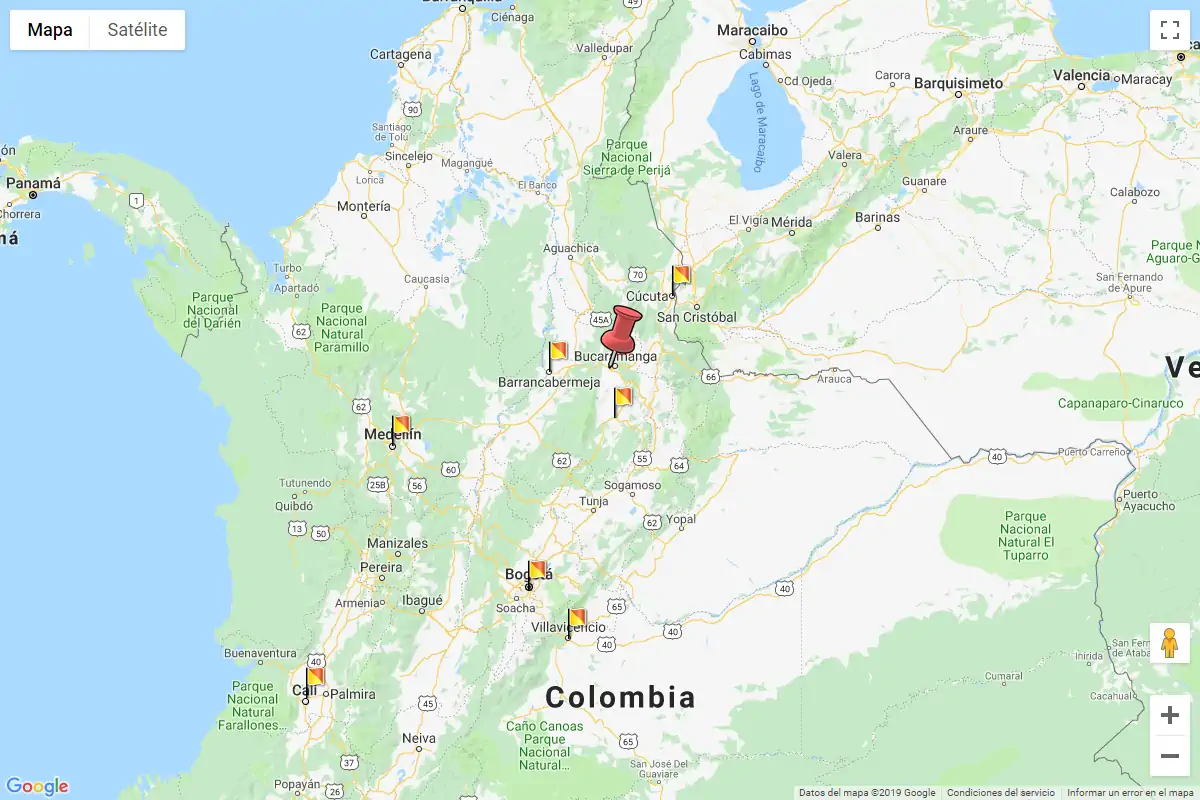Now, everything works as it should for now, the map works, the markers are in the correct location, but now, we will need to filter them according to our position (Bucaramanga) and according to a specific distance radius. We won't cover in this article how to filter them with JavaScript, but only filtering in the server side, so is up to you how to show them in the frontend later.

## 2. Understanding what we need to do

We will need to understand as first what we are going to do in the database and theorically. The following schema exposes 2 questions that we need to answer in order to accomplish the task, having in count that we will search through a circular area, defined by some distance that we will name Search radius: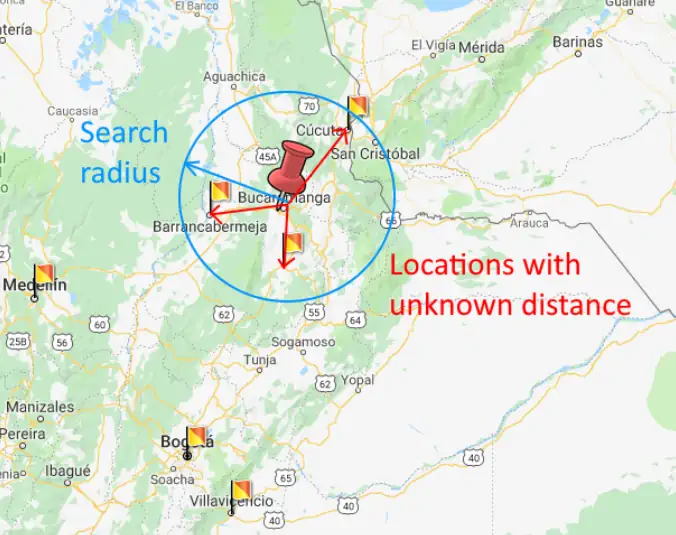Knowing that the distance between the initial location and the other coordinates is unknown:

• How can we define the search radius from the start point?
• How can we know when a location is inside the search radius?

Well, basically to define the search radius, you will be the one that will define a plain numeric value to this distance, for example we will use 150 kilometers! For our initial point we will use the coordinates of the city of Bucaramanga in Colombia that are in this example:

• lat: 7.08594109039762
• lng: 286.95225338731285

## 3. Preparing nearest locations query

Now, to answer the second question that we made previously of "How to know when a location is inside the search radius?", a location will be inside the radius as long as the distance between the initial point (Red Marker, Bucaramanga) and the location (Some Flag) is less than the defined search radius (150). So, how can we find the distance between the location and the initial point? For this, we will need to find the distance between coordinates. This is done through a lot of math, that we won't explain in this article, because otherwise it would get boring, so, we can resume it with a PHP function, that is way funier:

``````<?php

/**
* Method to find the distance between 2 locations from its coordinates.
*
* @param latitude1 LAT from point A
* @param longitude1 LNG from point A
* @param latitude2 LAT from point A
* @param longitude2 LNG from point A
*
* @return Float Distance in Kilometers.
*/
function getDistanceBetweenPointsNew(\$latitude1, \$longitude1, \$latitude2, \$longitude2, \$unit = 'Mi') {
\$theta = \$longitude1 - \$longitude2;

\$distance = acos(\$distance);
\$distance = \$distance * 60 * 1.1515;

switch(\$unit)
{
case 'Mi': break;
case 'Km' : \$distance = \$distance * 1.609344;
}

return (round(\$distance,2));
}``````

That's what we basically do to know the distance between 2 locations from their coordinates, for example the distance between Bucaramanga:

• lat: 7.08594109039762
• lng: 286.95225338731285

And Cúcuta:

• lat: 7.88475514
• lng: -72.49432589

Would be:

``````// Outputs: 107.76, distance in km between the given coordinates
echo getDistanceBetweenPointsNew(
7.08594109039762,
286.95225338731285,
7.88475514,
-72.49432589,
'Km'
);``````

Understanding this, we will do the same with SQL, this will provide us in the query with a new column namely distance that contains the value from the given coordinates. The SQL query to achieve this will be different if you are working in Kilometers or Miles:

### A. Searching in Kilometers

``````--- Where:
--- \$LATITUDE = the latitude of the start point e.g 7.08594109039762;
--- \$LONGITUDE = the longitude of the start point e.g 286.95225338731285;

SELECT * FROM (
SELECT *,
(
(
(
acos(
sin(( \$LATITUDE * pi() / 180))
*
sin(( `latitud_fieldname` * pi() / 180)) + cos(( \$LATITUDE * pi() /180 ))
*
cos(( `latitud_fieldname` * pi() / 180)) * cos((( \$LONGITUDE - `longitude_fieldname`) * pi()/180)))
) * 180/pi()
) * 60 * 1.1515 * 1.609344
)
as distance FROM `myTable`
) myTable
WHERE distance <= \$DISTANCE_KILOMETERS
LIMIT 15;``````

### B. Search in Miles

``````--- Where:
--- \$LATITUDE = the latitude of the start point e.g 7.08594109039762;
--- \$LONGITUDE = the longitude of the start point e.g 286.95225338731285;

SELECT * FROM (
SELECT *,
(
(
(
acos(
sin(( \$LATITUDE * pi() / 180))
*
sin(( `latitud_fieldname` * pi() / 180)) + cos(( \$LATITUDE * pi() /180 ))
*
cos(( `latitud_fieldname` * pi() / 180)) * cos((( \$LONGITUDE - `longitude_fieldname`) * pi()/180)))
) * 180/pi()
) * 60 * 1.1515
)
as distance FROM `myTable`
) myTable
WHERE distance <= \$DISTANCE_MILES
LIMIT 15;``````

## 4. Testing query

Now that we have the search radius distance, we can finally test our query replacing the values and simply running it (in the screenshot we used `140.85` instead of `150`, but is basically the same):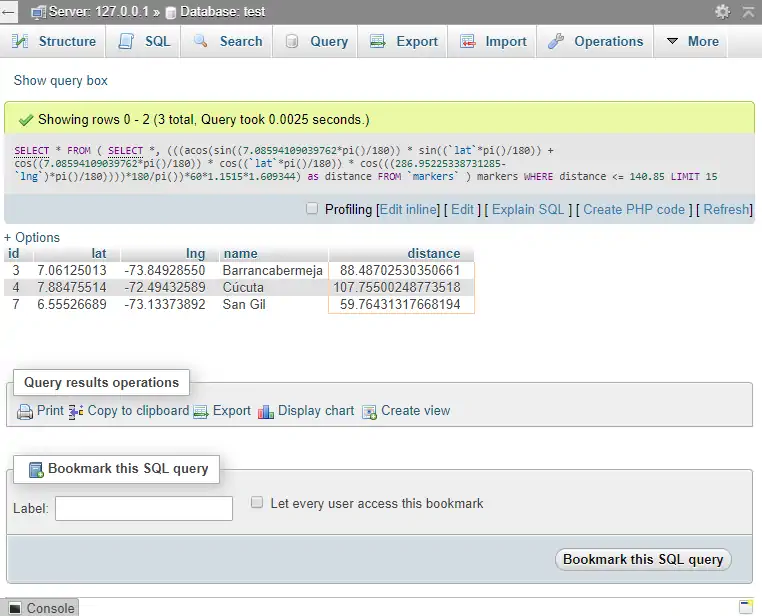Now with the given values, our query matched exactly a total of 3 locations that graphically and mathematically, match with our search. You can finally run the query with PHP, to handle the results as you want:

``````<?php
\$servername = "localhost";
\$dbname = "test";

// Create connection
mysqli_set_charset(\$conn,"utf8");
// Check connection
if (\$conn->connect_error) {
die("Connection failed: " . \$conn->connect_error);
}

// Bucaramanga Coordinates
\$lat = 7.08594109039762;
\$lon = 286.95225338731285;

// Only show places within 100km
\$distance = 150;

\$lat = mysqli_real_escape_string(\$conn, \$lat);
\$lon = mysqli_real_escape_string(\$conn, \$lon);
\$distance = mysqli_real_escape_string(\$conn, \$distance);

\$query = <<<EOF
SELECT * FROM (
SELECT *,
(
(
(
acos(
sin(( \$lat * pi() / 180))
*
sin(( `lat` * pi() / 180)) + cos(( \$lat * pi() /180 ))
*
cos(( `lat` * pi() / 180)) * cos((( \$lon - `lng`) * pi()/180)))
) * 180/pi()
) * 60 * 1.1515 * 1.609344
)
as distance FROM `markers`
) markers
WHERE distance <= \$distance
LIMIT 15;
EOF;

\$result = \$conn->query(\$query);

if (\$result->num_rows > 0) {
// output data of each row
while(\$row = \$result->fetch_assoc()) {
echo \$row["name"] . "<br>";
}
}

// Outputs:
// Barrancabermeja
// Cúcuta
// San Gil``````

Happy coding !